# Texas Go Math Grade 5 Lesson 12.1 Answer Key Cubes and Solid Figures

Refer to our Texas Go Math Grade 5 Answer Key Pdf to score good marks in the exams. Test yourself by practicing the problems from Texas Go Math Grade 5 Lesson 12.1 Answer Key Cubes and Solid Figures.

## Texas Go Math Grade 5 Lesson 12.1 Answer Key Cubes and Solid Figures

Essential Question
What is a unit cube and how can you use it to build a solid figure?
A rectangular prism is a solid figure with six rectangular faces, A unit cube is a cube with side lengths of 1 unit. A unit cube is said to have one cubic unit of volume and can be used to measure the volume of a solid figure. volume the amount of space inside a solid figure.

Investigate

You can build rectangular prisms using unit cubes. How many different rectangular prisms can you build with a given number of unit cubes?
Materialscentimeter cubes
A unit cube is a cube that has a length, width, and height of 1 unit. A cube has 6 square faces. All of its faces are congruent. It has 12 edges. The lengths of all its edges are equal.A. Build a rectangular prism with 2 unit cubes.
Think: When the 2 cubes are pushed together, the faces and edges that are pushed together make 1 face and 1 edge.
We know that,
In a unit cube,
There are 6 faces and 12 edges
So,
When we combine 2 unit cubes, it will become a single unit cube
A. How many faces does the rectangular prism have?
The number of faces a rectangular prism has is: 6 faces
B. How many edges does the rectangular prism have?
The number of edges a rectangular prism has is: 12 edgesB. Build as many different rectangular prisms as you can with 8 unit cubes.
The dimensions of the different rectangular prisms with 8 unit cubes is:
a. 2 units × 2 units × 2 units
b. 1 unit × 4 units × 2 units
c. 2 units × 4 units × 1 unit
d. 1 unit × 1 unit × 8 units

C. Record in units the dimensions of each rectangular prism you built with 8 cubes.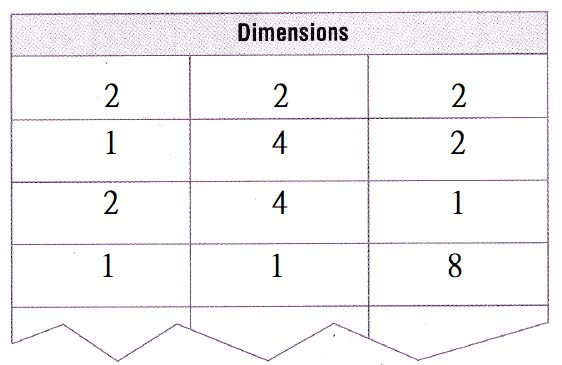So,
With 8 unit cubes, I can build 4 different rectangular prisms.

Math Talk
Mathematical Processes

Describe the different rectangular prisms that you can make 4 unit cubes?
The representation of the dimensions of the different rectangular prisms that you can make with 4 unit cubes is:
a. 2 units × 2 units × 1 unit
b. 1 unit × 4 units × 1 unit

Make Connections

You can build other solid figures and compare the solid figures by counting the number of unit cubes.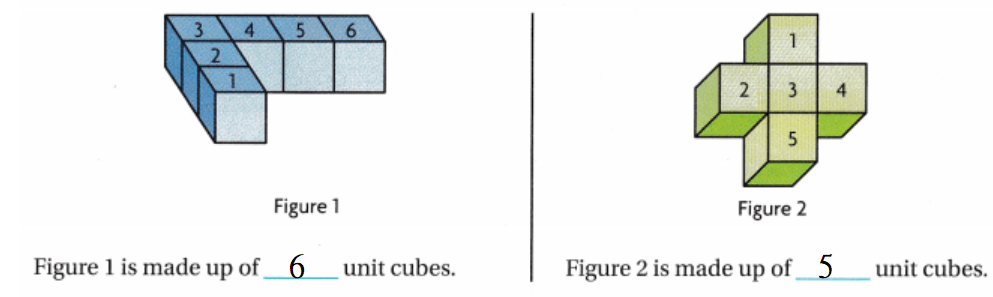So
Figure 1 has more unit cubes than Figure 2.

• Use 12 unit cubes to build a solid figure that is not a rectangular prism. Share your model with a partner. Describe how your model is the same and how it is different from your partner’s model.

Share and Show

Count the number of cubes used to build each solid figure.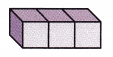Question 1.
The rectangular prism is made up of ___ unit cubes.
The given figure is:Now,
From the given figure,
We can observe that
There are 3 unit cubes
Hence, from the above,
We can conclude that
The rectangular prism is made up of 3 unit cubes.

Question 2.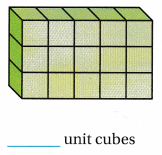The given figure is: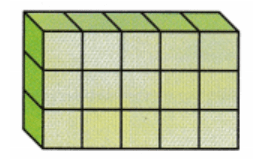Now,
From the given figure,
We can observe that
There are 15 unit cubes
Hence, from the above,
We can conclude that
The rectangular prism is made up of 15 unit cubes

Question 3.The given figure is: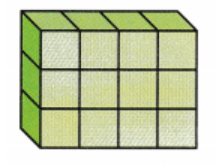Now,
From the given figure,
We can observe that
There are 12 unit cubes
Hence, from the above,
We can conclude that
The rectangular prism is made up of 12 unit cubes

Question 4.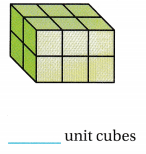The given figure is: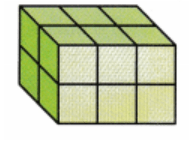Now,
From the given figure,
We can observe that
There are 6 unit cubes
Hence, from the above,
We can conclude that
The rectangular prism is made up of 6 unit cubes

Question 5.The given figure is:Now,
From the given figure,
We can observe that
There are 5 unit cubes
Hence, from the above,
We can conclude that
The rectangular prism is made up of 5 unit cubes

Question 6.The given figure is: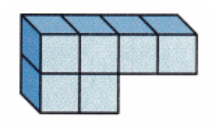Now,
From the given figure,
We can observe that
There are 6 unit cubes
Hence, from the above,
We can conclude that
The rectangular prism is made up of 6 unit cubes

Question 7.The given figure is:Now,
From the given figure,
We can observe that
There are 7 unit cubes
Hence, from the above,
We can conclude that
The rectangular prism is made up of 7 unit cubes

Problem Solving

Question 8.
H.O.T. How are the rectangular prisms in Exercises 3 and 4 related? Is there a different rectangular prism with the same relationship? Use a model or a diagram to show your answer.
The rectangular prisms in Exercise 3 and Exercise 4 are respectively:Now,
From the above rectangular prisms,
We can observe that
In Exercises 3 and 4,
The number of rows is the same
The number of columns in Exercise 3 is: 5
The number of columns in Exercise 4 is: 4
Hence, from the above,
We can conclude that
The number of rows in Exercise 3 and Exercise 4 is the same
There are no different rectangular prisms with the same relationship

Question 9.
Write Math Explain why a rectangular prism composed of 2 unit cubes has 6 faces. How do its dimensions compare to a unit cube?
We know that,
A unit cube consists of 6 faces and 12 edges
Now,
When we combine 2 unit cubes to make a rectangular prism, the combined 2 units will become a large unit cube
So,
The number of faces will remain the same i.e., 6 faces
Hence, from the above,
We can conclude that
The rectangular prism with 2 unit cubes has 6 faces and its dimensions will be greater when compared to the dimensions of a unit cube

Question 10.
Write Math Explain how the number of edges for the rectangular prism compares to the number of edges for the unit cube.
We know that,
In a unit cube,
The number of faces is: 6
The number of edges is: 12
Now,
When we observe the above,
We can say that
The number of edges = 2 × The number of faces
Hence, from the above,
We can conclude that
The number of edges for the rectangular prism when compare to the number of edges for the unit cube is:
The number of edges = 2 × The number of faces

Question 11.
Multi-Step The Nakagin Capsule Tower has 140 modules and is 14 stories high. If all of the modules were divided evenly among the number of stories, how many modules would be on each floor? How many different rectangular prisms could be made from that number?It is given that
The Nakagin Capsule Tower has 140 modules and is 14 stories high. If all of the modules were divided evenly among the number of stories
Now,
According to the given information,
The number of modules on each floor = (The total number of modules) ÷ (The total number of stories)
= latex]\frac{140}{14}[/latex]
= 10 modules
Now,
The number of rectangular prisms from 10 modules = 2 × 5 (or) 5 × 2 (or) 1 × 10 (or) 10 × 1 rectangular prisms
Hence, from the above,
We can conclude that
The number of modules on each floor is: 10 modules
The number of rectangular prisms from 10 modules is: 2 × 5 (or) 5 × 2 (or) 1 × 10 (or) 10 × 1 rectangular prisms

Question 12.
A cube-shaped house can hold 1,000 unit cubes that are 1 meter by 1 meter by 1 meter. Describe the dimensions of the house using unit cubes.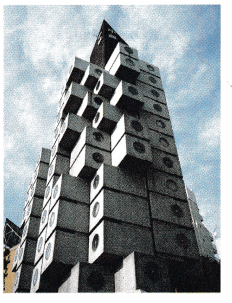It is given that
A cube-shaped house can hold 1,000 unit cubes that are 1 meter by 1 meter by 1 meter
Now,
The dimensions of the house using the unit cubes = 2 × 500 (or) 500 × 2 (or) 1 × 1,000 (or) 1,000 × 1
Hence, from the above,
We can conclude that
The dimensions of the house using the unit cubes is: 2 × 500 (or) 500 × 2 (or) 1 × 1,000 (or) 1,000 × 1 rectangular prisms

Question 13.
Multi-Step Seth makes 3 more of the figure shown below to build a fort. How many cubes does he use?(A) 5 cubes
(B) 25 cubes
(C) 4 cubes
(C) 20 cubes
It is given that
Seth makes 3 more of the figure shown below to build a fort
Now,
The given figure is:Now,
The total number of cubes needed to build a fort = (The number of cubes present in the given figure) × 4
= 5 × 4
= 20 cubes
Hence, from the above,
We can conclude that
The total number of cubes needed to build a fort is:Question 14.
How many cubes were used to make the figure?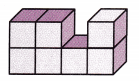(A) 7 cubes
(B) 9 cubes
(C) 8 cubes
(C) 10 cubes
The given figure is:Now,
From the given figure,
We can observe that
There are 7 cubes
Hence, from the above,
We can conclude that
The number of cubes that were used to make the given figure is:Question 15.
Multi-Step Alexandra built these two figures. How many cubes did she use in all?
(A) 9 cubes
(B) 6 cubes
(C) 15 cubes
(D) 30 cubes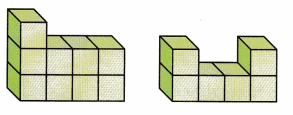It is given that
Alexandra built the two figures.
Now,
The given figures are:Now,
From the given figures,
We can observe that
The total number of cubes = 9 + 6
= 15 cubes
Hence, from the above,
We can conclude that
The number of cubes did Alexandria used are: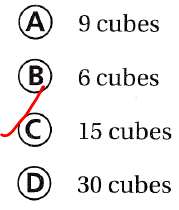Texas Test Prep

Question 16.
Multi-Step How many more cubes can Marcy use to make the figure below a rectangular prism?
(A) 5 cubes
(B) 13 cubes
(C) 2 cubes
(D) 7 cubesThe given figure is:Now,
To make the given figure a complete rectangular prism,
We have to add 2 more unit cubes
Hence, from the above,
We can conclude that
The number of more cubes Marcy can use to make the figure below a rectangular prism is: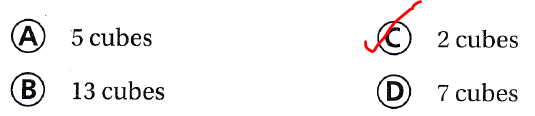### Texas Go Math Grade 5 Lesson 12.1 Homework and Practice Answer Key

Count the number of cubes used to build each solid figure.

Question 1.The given figure is: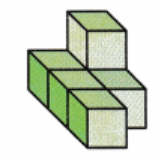Now,
From the given figure,
We can observe that
There are 5 unit cubes
Hence, from the above,
We can conclude that
The rectangular prism is made up of 5 unit cubes

Question 2.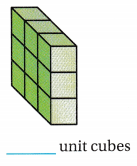The given figure is:Now,
From the given figure,
We can observe that
There are 12 unit cubes
Hence, from the above,
We can conclude that
The rectangular prism is made up of 12 unit cubes

Question 3.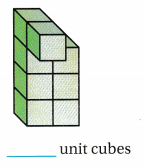The given figure is: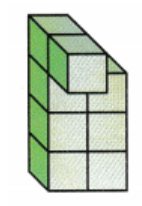Now,
From the given figure,
We can observe that
There are 8 unit cubes
Hence, from the above,
We can conclude that
The rectangular prism is made up of 8 unit cubes

Question 4.The given figure is:Now,
From the given figure,
We can observe that
There are 4 unit cubes
Hence, from the above,
We can conclude that
The rectangular prism is made up of 4 unit cubes

Question 5.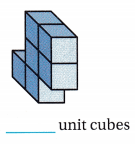The given figure is: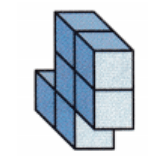Now,
From the given figure,
We can observe that
There are 6 unit cubes
Hence, from the above,
We can conclude that
The rectangular prism is made up of 6 unit cubes

Question 6.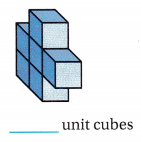The given figure is: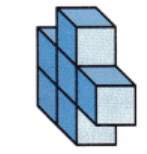Now,
From the given figure,
We can observe that
There are 5 unit cubes
Hence, from the above,
We can conclude that
The rectangular prism is made up of 5 unit cubes

Question 7.The given figure is:Now,
From the given figure,
We can observe that
There are 12 unit cubes
Hence, from the above,
We can conclude that
The rectangular prism is made up of 12 unit cubes

Question 8.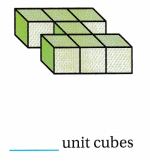The given figure is: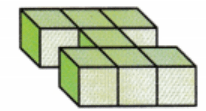Now,
From the given figure,
We can observe that
There are 7 unit cubes
Hence, from the above,
We can conclude that
The rectangular prism is made up of 7 unit cubes

Question 9.The given figure is: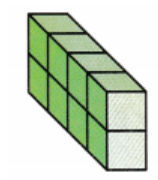Now,
From the given figure,
We can observe that
There are 8 unit cubes
Hence, from the above,
We can conclude that
The rectangular prism is made up of 8 unit cubes

Problem Solving

Question 10.
Jen used unit cubes to build a rectangular prism with a length of 4 cubes, a width of 3 cubes, and a height of 5 cubes. Ethan built a rectangular prism with a length of 3 cubes, a width of 3 cubes, and a height of 6 cubes. Who used more cubes? Explain.
It is given that
Jen used unit cubes to build a rectangular prism with a length of 4 cubes, a width of 3 cubes, and a height of 5 cubes. Ethan built a rectangular prism with a length of 3 cubes, a width of 3 cubes, and a height of 6 cubes
Now,
According to the given information,
The number of cubes used by Jen to build a rectangular prism = 4 × 3 × 5
= 60 unit cubes
The number of cubes used by Ethan to build a rectangular prism = 3 × 3 × 6
= 54 unit cubes
Hence, from the above,
We can conclude that
Jen used more cubes

Question 11.
Mrs. Hernandez keeps her classroom supply of centimeter cubes in a box that is 20 cm by 6 cm by 3 cm. How many centimeter cubes will fill the box?
It is given that
Mrs. Hernandez keeps her classroom supply of centimeter cubes in a box that is 20 cm by 6 cm by 3 cm
Now,
According to the given information,
The number of centimeter cubes that will fill the box = 20 × 6 × 3
= 360 centimeter cubes
Hence, from the above,
We can conclude that
The number of centimeter cubes that will fill the box is: 360 centimeter cubes

Lesson Check

Texas Test Prep

Question 12.
Blame makes the figure shown below using unit cubes. How many cubes does Blame use?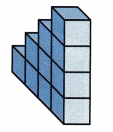(A) 14 cubes
(B) 20 cubes
(C) 10 cubes
(D) 9 cubes
It is given that
Blame makes the figure shown below using unit cubes
Now,
The given figure is:Now,
From the given figure,
We can observe that
The number of cubes present in the given rectangular prism is: 10 cubes
Hence, from the above,
We can conclude that
The number of cubes Blaine did use is: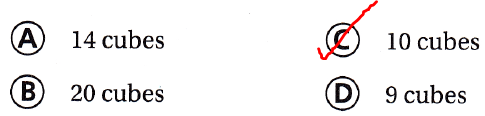Question 13.
Lucas covers a rectangle with 5 rows of 4 unit cubes. Then he stacks identical layers to make a prism. How many cubes does Lucas use to make 4 layers?
(A) 80 cubes
(B) 20 cubes
(C) 100 cubes
(D) 24 cubes
It is given that
Lucas covers a rectangle with 5 rows of 4 unit cubes. Then he stacks identical layers to make a prism.
Now,
According to the given information,
The number of cubes does Lucas use to make 4 layers = (5 × 4) × 4
= 20 × 4
= 80 cubes
Hence, from the above,
We can conclude that
The number of cubes does Lucas use to make 4 layers is: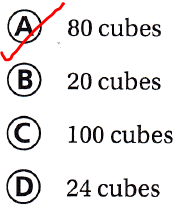Question 14.
Lola has 30 cubes. She wants to use all the cubes to make a rectangular prism. Which could be the dimensions of her prism?
(A) 5 × 2 × 3
(B) 3 × 3 × 3
(C) 4 × 5 × 2
(D) 3 × 10 × 2
It is given that
Lola has 30 cubes
Now,
According to the given information,
The dimensions of Lola’s prism = 10 × 3 × 1 (or) 5 × 6 × 1 (or) 5 × 3 × 2 (or) 5 × 2 × 3
Hence, from the above,
We can conclude that
The dimensions of Lola’s prism is: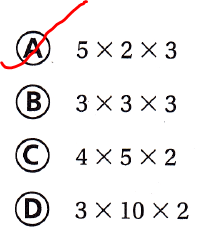Question 15.
Multi-Step How many cubes will be in the figure shown below if 12 cubes are added?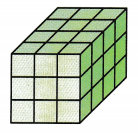(A) 36 cubes
(B) 24 cubes
(C) 48 cubes
(D) 60 cubes
The given figure is:Now,
From the given figure,
We can observe that
The number of cubes are: 12
Now,
The total number of cubes = 12 + 12
= 24 cubes
Hence, from the above,
We can conclude that
The total number of cubes in the given figure after 12 cubes are added is: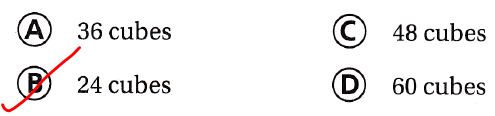Question 16.
Multi-Step Stella built these two figures. How many more cubes did she use in figure A than in figure B?(A) 8 cubes
(B) 5 cubes
(C) 3 cubes
(D) 2 cubes
It is given that
Stella built these two figures
Now,
The given figures are:Now,
From the given figures,
We can observe that
The difference between the number of cubes between Figure A and Figure B = 8 – 5
= 3 cubes
Hence, from the above,
We can conclude that
The number of more cubes that Stella used in Figure A than in Figure B is:Question 17.
Multi-Step Owen wants to make a rectangular prism with a length of 6 cubes, a width of 3 cubes, and a height of 4 cubes. He has 54 cubes. How many more cubes does Owen need?
(A) 36 cubes
(B) 42 cubes
(C) 22 cubes
(D) 18 cubes